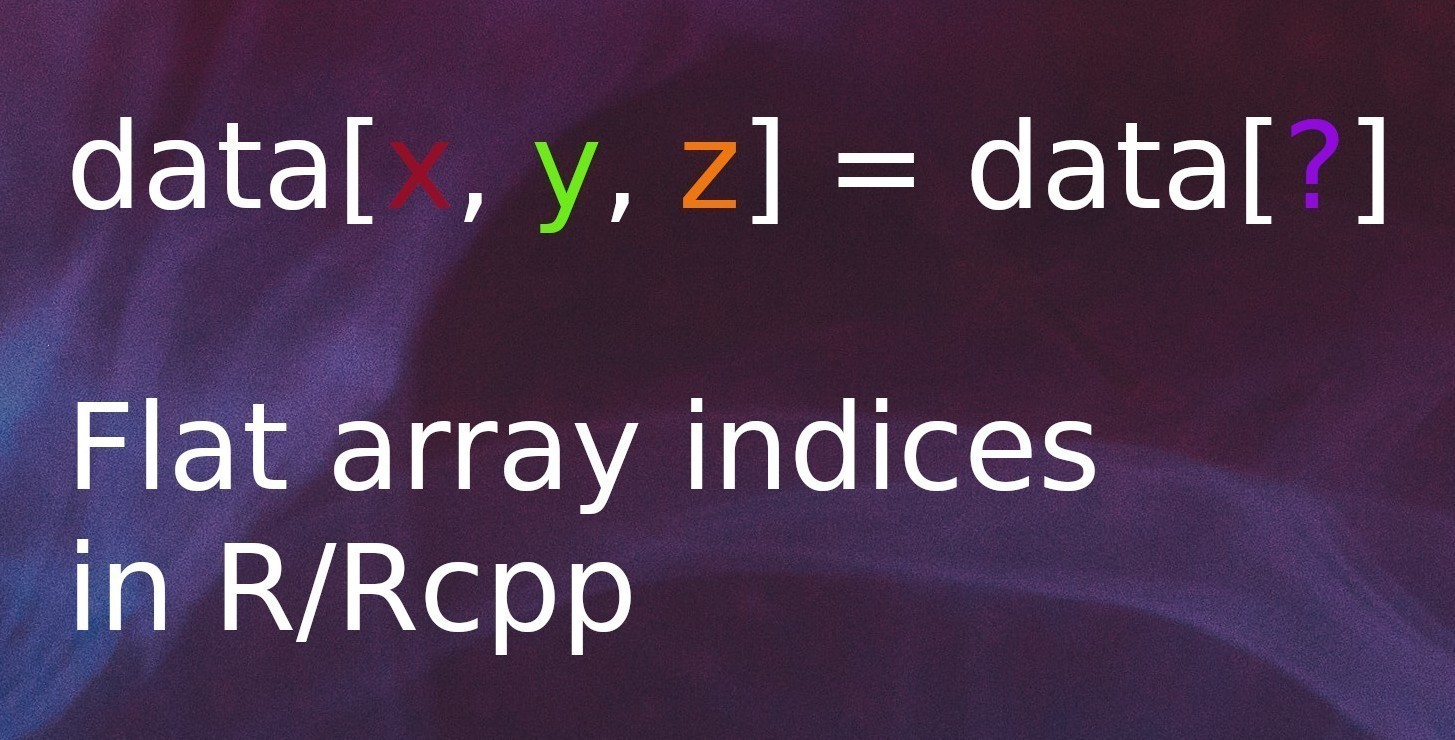[This article was first published on R on krzjoa, and kindly contributed to R-bloggers]. (You can report issue about the content on this page here)
Want to share your content on R-bloggers? click here if you have a blog, or here if you don't.Although 3-dimensional arrays are not the most common object used among the R projects, which are dominated by data.frame-like objects. However, when we’re starting to work with deep learning, (e.g. using {keras}), we can run into such objects many times, especially in fields like time series forecasting or NLP.

The question I’d like to answer in this post is how to find ‘flat’ equivalent of the three-element index for the 3-dimensional arrays.

## Problem

Let’s create a sample array to present the problem.

data <- array(0, c(20, 7, 5))


To get a single element, an array can be subscripted in two ways:

• using indices for all the existing dimensions
data[12, 3, 1] <- 7
data[12, 3, 1]

##  7

• using a single index

In this approach the array is treated as a flat vector, so I named this kind of indexing flat index.

data <- 8
data

##  8


But how we can easily tranform the first type of indexing into the second one?

## Solution

The solution generalized to the $n$-dimensional case can be expressed as:

$[x_1, x_2, x_3, ..., x_n] = x_1 + \sum_{i=2}^{n}x_i\prod_{j = 1}^{i-1}d_j$

where $x_i$ means i-th index and $d_i$ i-th dimension size. This solution takes into account the 1-based indexing which is used in R.

## Example

Suppose we have an array with the same dimesnions as shown above: $(20, 7, 5)$. We’d like to access an element at index $(11, 3, 2)$.

example <- array(0, c(20, 7, 5))
example[11, 3, 2] <- 7


We calculate the flat index according to the aforementioned schema.

flat_idx <- 11 + (3 - 1) * 20 + (2 - 1) * 20 * 7
example[flat_idx]

##  7


## Code snippets

In R code;

#' Get an index you can use access an array element at once
#' [x, y, z] = x + (y - 1) * x_dim + (z - 1) * x_dim * y_dim
#' [x, y] = x + (y-1) * x_dim
#'
#' @param dim_sizes Dimensions sizes
#' @param dim_indices Indices
flat_index <- function(dim_sizes, dim_indices){
dim_indices + sum((dim_indices[-1] - 1) * cumprod(dim_sizes[-length(dim_sizes)]))
}

# Example 1
arr <- array(0, c(4,5,6,7))
arr[1,2,3,4] <- 777

flat_index(c(4,5,6,7), c(1,2,3,4))

##  405

which(arr == 777)

##  405

# Example 2
arr2 <- array(0, c(32,10,5))
arr2[12,8,4] <- 777

flat_index( c(32,10,5), c(12,8,4))

##  1196

which(arr2 == 777)

##  1196


In Rcpp, you can use the following code snippet (for 3-dimensional arrays):

// In C++ transformed to the zero-based index
int flat_index(int dim1, int dim2,
int idx1, int idx2, int idx3){
return idx1 + idx2 * dim1 + idx3 * dim1 * dim2;
}